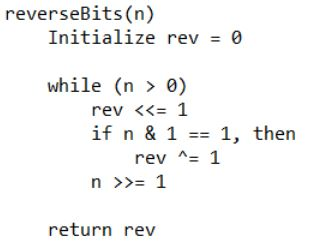Skip to content
Related Articles
Reverse actual bits of the given number
• Difficulty Level : Medium
• Last Updated : 08 Apr, 2021

Given a non-negative integer n. The problem is to reverse the bits of n and print the number obtained after reversing the bits. Note that the actual binary representation of the number is being considered for reversing the bits, no leading 0’s are being considered.
Examples :

```Input : 11
Output : 13
(11)10 = (1011)2.
After reversing the bits we get:
(1101)2 = (13)10.

Input : 10
Output : 5
(10)10 = (1010)2.
After reversing the bits we get:
(0101)2 = (101)2
= (5)10.```

In this approach, one by one bits in binary representation of n are being obtained with the help of bitwise right shift operation and they are being accumulated in rev with the help of bitwise left shift operation.
Algorithm:## C++

 `// C++ implementation to reverse bits of a number``#include ` `using` `namespace` `std;` `// function to reverse bits of a number``unsigned ``int` `reverseBits(unsigned ``int` `n)``{``    ``unsigned ``int` `rev = 0;``    ` `    ``// traversing bits of 'n' from the right``    ``while` `(n > 0)``    ``{``        ``// bitwise left shift``        ``// 'rev' by 1``        ``rev <<= 1;``        ` `        ``// if current bit is '1'``        ``if` `(n & 1 == 1)``            ``rev ^= 1;``        ` `        ``// bitwise right shift``        ``// 'n' by 1``        ``n >>= 1;``            ` `    ``}``    ` `    ``// required number``    ``return` `rev;``}` `// Driver program to test above``int` `main()``{``    ``unsigned ``int` `n = 11;``    ``cout << reverseBits(n);``    ``return` `0;``}`

## Java

 `// Java implementation to``// reverse bits of a number``class` `GFG``{``    ``// function to reverse bits of a number``    ``public` `static` `int` `reverseBits(``int` `n)``    ``{``        ``int` `rev = ``0``;` `        ``// traversing bits of 'n'``        ``// from the right``        ``while` `(n > ``0``)``        ``{``            ``// bitwise left shift``            ``// 'rev' by 1``            ``rev <<= ``1``;` `            ``// if current bit is '1'``            ``if` `((``int``)(n & ``1``) == ``1``)``                ``rev ^= ``1``;` `            ``// bitwise right shift``            ``//'n' by 1``            ``n >>= ``1``;``        ``}``        ``// required number``        ``return` `rev;``    ``}` `    ``// Driver code``    ``public` `static` `void` `main(String[] args)``    ``{``        ``int` `n = ``11``;``        ``System.out.println(reverseBits(n));``    ``}``}` `// This code is contributed``// by prerna saini.`

## Python3

 `# Python 3 implementation to``# reverse bits of a number`  `# function to reverse``# bits of a number``def` `reverseBits(n) :``    ` `    ``rev ``=` `0``    ` `    ``# traversing bits of 'n' from the right``    ``while` `(n > ``0``) :``        ` `        ``# bitwise left shift 'rev' by 1``        ``rev ``=` `rev << ``1``        ` `        ``# if current bit is '1'``        ``if` `(n & ``1` `=``=` `1``) :``            ``rev ``=` `rev ^ ``1``        ` `        ``# bitwise right shift 'n' by 1``        ``n ``=` `n >> ``1``        ` `    ` `    ``# required number``    ``return` `rev``    ` `# Driver code``n ``=` `11``print``(reverseBits(n))`  `# This code is contributed``# by Nikita Tiwari.`

## C#

 `// C# implementation to``// reverse bits of a number``using` `System;``class` `GFG``{``    ``// function to reverse bits of a number``    ``public` `static` `int` `reverseBits(``int` `n)``    ``{``        ``int` `rev = 0;` `        ``// traversing bits of 'n'``        ``// from the right``        ``while` `(n > 0)``        ``{``            ``// bitwise left shift``            ``// 'rev' by 1``            ``rev <<= 1;` `            ``// if current bit is '1'``            ``if` `((``int``)(n & 1) == 1)``                ``rev ^= 1;` `            ``// bitwise right shift``            ``//'n' by 1``            ``n >>= 1;``        ``}``        ``// required number``        ``return` `rev;``    ``}` `    ``// Driver code``    ``public` `static` `void` `Main()``    ``{``        ``int` `n = 11;``        ``Console.WriteLine(reverseBits(n));``    ``}``}` `// This code is contributed``// by vt_m.`

## PHP

 ` 0)``    ``{``        ``// bitwise left shift``        ``// 'rev' by 1``        ``\$rev` `<<= 1;``        ` `        ``// if current bit is '1'``        ``if` `(``\$n` `& 1 == 1)``            ``\$rev` `^= 1;``        ` `        ``// bitwise right shift``        ``// 'n' by 1``        ``\$n` `>>= 1;``            ` `    ``}``    ` `    ``// required number``    ``return` `\$rev``;``}` `// Driver code``\$n` `= 11;``echo` `reverseBits(``\$n``);` `// This code is contributed by mits``?>`

## Javascript

 ``

Output :

`13`

Time Complexity: O(num), where num is the number of bits in the binary representation of n.

Attention reader! Don’t stop learning now. Get hold of all the important DSA concepts with the DSA Self Paced Course at a student-friendly price and become industry ready.  To complete your preparation from learning a language to DS Algo and many more,  please refer Complete Interview Preparation Course.

In case you wish to attend live classes with industry experts, please refer Geeks Classes Live

My Personal Notes arrow_drop_up